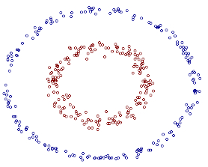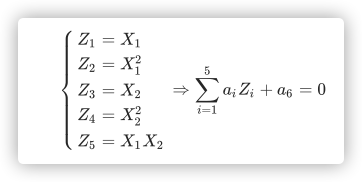核方法0x01 为什么需要核函数

SVM显然是线性分类器，但数据如果根本就线性不可分怎么办？0x02 什么是核函数

引入:

$a_1X_1+a_2X_1^2+a_3X_2+a_4X_2^2+a_5X_1X_2+a_6=0$$\sum_{i=1}^5 a_iZ_i +a_6=0$

核函数的定义：

$\mathcal{X}$是输入空间(欧式空间$R^n$的子集或离散集合)，又设$\mathcal{H}$为特征空间(希尔伯特空间)，如果存在一个从$\mathcal{X}$$\mathcal{H}$的映射：

$\phi(x):\mathcal{X} \rightarrow \mathcal{H}$

$K(x,z) = \phi(x)\cdot\phi(z)$

映射函数$\phi$的取法并不是唯一的：

$(x\cdot z)^2 = (x^{(1)}z^{(1)}+x^{(2)}z^{(2)})^2 = (x^{(1)}z^{(1)})^2+2x^{(1)}z^{(1)}x^{(2)}z^{(2)}+(x^{(2)}z^{(2)})^2$

0x03 正定核

预备知识：

• 首先定义映射$\phi$并构成向量空间$\mathcal{S}$.
• $\mathcal{S}$定义内积构成内积空间.
• $\mathcal{S}$完备化成希尔伯特空间.

1.定义映射，构成向量空间$\mathcal{S}$.

$\phi：x \rightarrow K(\cdot,x)$

$f(\cdot)=\sum_{i=1}^m\alpha_iK(\cdot,x_i) \tag{1}$

2.在$\mathcal{S}$定义内积构成内积空间.

$\mathcal{S}$上定义一个运算$*$,对任意的$f,g\in \mathcal{S}$,

$g(\cdot)=\sum_{j=1}^l\beta_jK(\cdot,z_j) \tag{2}$

$f*g = \sum_{i=1}^m\sum_{j=1}^l\alpha_i\beta_jK(x_i.z_j) \tag{3}$

$\begin{array}{l} a.&(cf)*g=c(f*g),c\in R\\ b.&(f+g)*h=f*h+g*h,h\in \mathcal{S}\\ c.&f*g=g*f\\ d.&f*f\geq0,f*f=0 ⇔ f=0 \end{array}$

$f*f=\sum_{i,j=1}^m\alpha_i\alpha_jK(x_i.x_j)$

$|f*g|^2\leq(f*f)(g*g) \tag{4}$

$f,g\in \mathcal{S},\lambda \in R$,则$f+\lambda g\in\mathcal{S}$,于是：

$(f+\lambda g)(f+\lambda g)\geq0\\ f*f+2\lambda(f*g)+\lambda^2(g*g)\geq0$

$(f*g)^2-(f*f)(g*g)\leq0$

$g=K(\cdot,x)$，则由式(3)可得：$K(\cdot,x)*f=\sum_{i=1}^m\alpha_iK(x,x_i)=f(x)$.所以有：

$|f(x)|^2=|K(\cdot,x)*f|^2 \tag{5}$

$|K(\cdot,x)*f|^2\leq(K(\cdot,x)*K(\cdot,x))(f*f)=K(x,x)(f*f)$

$|f(x)|^2\leq K(x,x)(f*f)$

$f\cdot g = \sum_{i=1}^m\sum_{j=1}^l\alpha_i\beta_jK(x_i.z_j) \tag{6}$

3.将$\mathcal{S}$完备化成希尔伯特空间.

$||f||=\sqrt{f\cdot f}$

充要条件：

$K:\mathcal{X}×\mathcal{X}\rightarrow R$是对称函数，则$K(x,z)$为正定核函数的充要条件是对任意的$x_i\in \mathcal{X},i=1,2,3,...,m,K(x,z)$对应的$Gram$矩阵：

$K=[K(x_i,x_j)]_{m×m}$

• 必要性：

由于$K(x,z)$$\mathcal{X}×\mathcal{X}$上的正定核，所以存在从$\mathcal{X}\rightarrow \mathcal{H}$的映射$\phi$，使得$K(x,z)=\phi(x)\cdot \phi(z)$.

构造Gram矩阵：

$[K_{ij}]_{m×m}=[K(x_i,x_j)]_{m×m}$

对任意的$c_1,c_2,...,c_m \in R$,有：

$\begin{array}{l} \sum_{i,j=1}^mc_ic_jK(x_i,x_j)&=\sum_{i,j=1}^mc_ic_j(\phi(x)\cdot \phi(z))\\ &=\left( \sum_ic_i\phi(x_i)\right)\cdot\left( \sum_jc_j\phi(x_j)\right)\\ &=||\sum_ic_i\phi(x_i)||^2 \geq 0 \end{array}$

• 充分性：

假设$K(x,z)$是定义在$\mathcal{X}×\mathcal{X}$上的对称函数，对任意的$x_i\in \mathcal{X},i=1,2,3,...,m,K(x,z)$关于$x_1,x_2,...,x_m$的Gram矩阵是半正定的。可以构造映射函数$\phi:x\rightarrow K(\cdot,x)$.

$K(\cdot,x)*f=\sum_{i=1}^m\alpha_iK(x,x_i)=f(x)$

并且$K(\cdot,x)\cdot K(\cdot,z)=K(x,z)$可知：$K(x,z)=\phi(x)\cdot\phi(z)$

表明$K(x,z)$是核函数。

0x04 常见核函数

• 线性核函数

$K(x,z) = x\cdot z$

线性核，主要用于线性可分的情况，我们可以看到特征空间到输入空间的维度是一样的，其参数少速度快，对于线性可分数据，其分类效果很理想，因此我们通常首先尝试用线性核函数来做分类，看看效果如何，如果不行再换别的。

• 多项式核函数

$K(x,z) = (x\cdot z+1)^p$

多项式核函数可以实现将低维的输入空间映射到高纬的特征空间，但是多项式核函数的参数多，当多项式的阶数比较高的时候，核矩阵的元素值将趋于无穷大或者无穷小，计算复杂度会大到无法计算。

• 高斯核函数

$K(x,z) = exp(-\frac{||x-z||^2}{2\sigma^2})$

高斯径向基函数是一种局部性强的核函数，其可以将一个样本映射到一个更高维的空间内，该核函数是应用最广的一个，无论大样本还是小样本都有比较好的性能，而且其相对于多项式核函数参数要少，因此大多数情况下在不知道用什么核函数的时候，优先使用高斯核函数。

• Sigmoid核函数

$K(x,z) = tanh(\gamma x\cdot z+\theta)$

采用sigmoid核函数，支持向量机实现的就是一种多层神经网络。

• 如果特征的数量大到和样本数量差不多，则选用LR或者线性核的SVM；
• 如果特征的数量小，样本的数量正常，则选用SVM+高斯核函数；
• 如果特征的数量小，而样本的数量很大，则需要手工添加一些特征从而变成第一种情况。